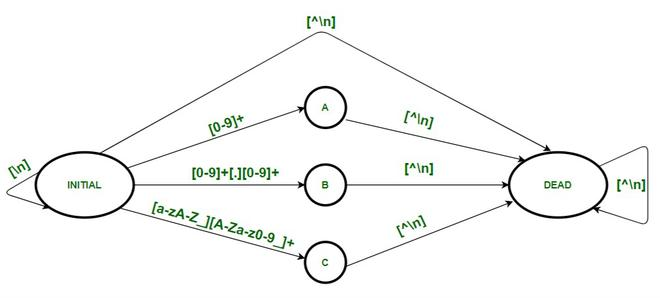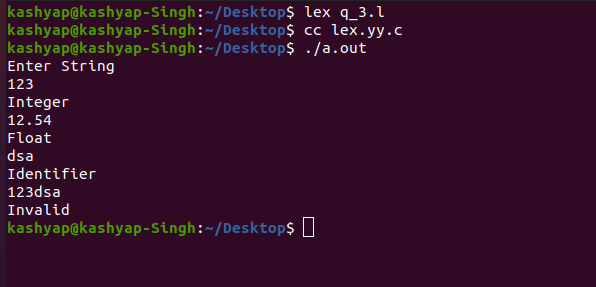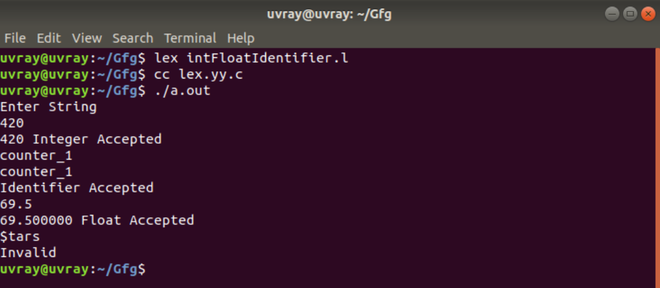Open in App
Not now

# LEX Code to Identify and print Integer & Float Constants and Identifier

• Difficulty Level : Hard
• Last Updated : 26 Sep, 2022

In this article, we will discuss how you can solve the problem, and also you will see how you can design problems related to DFA in LEX Code to Identify and print Integer & Float Constants and identifiers. Let’s discuss it one by one.

Problem Overview :
Design a DFA in LEX Code to Identify and print Integer & Float Constants and identifiers.

Note –
Regular Expression for Integer, float, and Identifier is as follows.

```Integer    - [0-9]+
Float      - [0-9]+[.][0-9]+
Identifier - [A-Za-z_][A-Za-z0-9_]+```

Example –

```Input  : 35
Output : Integer

Input  : 3.98
Output : Float

Input  : kashyap
Output : Identifier

Input  : 123Singh
Output : Invalid```

Approach :
LEX provides us with an INITIAL state by default. So to make a DFA, use this initial state as the initial state of the DFA. Define four more states A, B, C, and DEAD. DEAD is the dead state that would be used if an invalid or wrong input is encountered and it prints “Invalid”. A is used when an integer is encountered, which prints “Integer” and B is used when a floating constant is encountered which prints “Float” and C is used when an identifier is encountered, which prints “Identifier”.  A new line character (\n) marks the end of the input so transition to the INITIAL state and print the output or print “Not Accepted” or “Invalid.”Note:-
To compile the lex program we need to have a Unix system that has flex installed into it. Then we need to save the file with .l extension. For Example- filename.l Then after saving the program closes the lex file and then open the terminal and write the following commands.

```lex filename.l
cc lex.yy.c
./a.out```

LEX Code –

## C

 `// Declaration Section` `%{` `%}`   `%s A B C DEAD        ``// Declaring states` `  `  `// Rules Section` `%%` `[0-9]+ BEGIN A;` `[0-9]+[.][0-9]+ BEGIN B;` `[A-Za-z_][A-Za-z0-9_]* BEGIN C;` `[^\n] BEGIN DEAD;` `\n BEGIN INITIAL; {``printf``(``"Not Accepted\n"``);}`   `[^\n] BEGIN DEAD;` `\n BEGIN INITIAL; {``printf``(``"Integer\n"``);}`   `[^\n] BEGIN DEAD;` `\n BEGIN INITIAL; {``printf``(``"Float\n"``);}`   `[^\n] BEGIN DEAD;` `\n BEGIN INITIAL; {``printf``(``"Identifier\n"``);}`     `[^\n] BEGIN DEAD;` `\n BEGIN INITIAL; {``printf``(``"Invalid\n"``);} `   `%%` `  `  `// Auxiliary Functions` `int` `yywrap()` `{` ` ``return` `1;` `}`   `int` `main()` `{` ` ``printf``(``"Enter String\n"``);` ` ``yylex();` ` ``return` `0;` `}`

Output :#### Method 2

If you want to print the identified tokens as well then you can apply the following Lex code to print the matched integer, float and identifier.

LEX Code-

## C

 `//Declaration Section` `%{` `#include` `int` `num_int;            ``// stores integer` `char` `*str;                ``// stores identifier` `double` `num_float;        ``// stores float` `%}`   `%s A B C DEAD        ``//Declaring States`   `//Rules Section` `%%` ` [0-9]+ BEGIN A;                    {num_int = ``atoi``(yytext);}` ` [0-9]+``"."``[0-9]+ BEGIN B;            {num_float = ``atof``(yytext);}            ` ` [a-zA-Z_][a-zA-Z0-9_]* BEGIN C;    {str = yytext;}` ` [^\n] BEGIN DEAD;` ` [\n] BEGIN INITIAL;        {``printf``(``"Not Accepted\n"``);}`   ` [^\n] BEGIN DEAD;` ` \n BEGIN INITIAL;        {``printf``(``"%d Integer Accepted\n"``, num_int);}`   ` [^\n] BEGIN DEAD;` ` \n BEGIN INITIAL;        {``printf``(``"%lf Float Accepted\n"``, num_float);}`   ` [^\n] BEGIN DEAD;` ` \n BEGIN INITIAL;        {``printf``(``"%sIdentifier Accepted\n"``, str);}`   ` [^\n] BEGIN DEAD;` ` \n BEGIN INITIAL;    {``printf``(``"Invalid\n"``);}` `%%`   `//Auxiliary Functions` `int` `yywrap()` `{` `      ``return` `1;` `}`   `int` `main(){` `    ``printf``(``"Enter String:\n"``);` `     ``yylex();` `     ``return` `0;` `}`

Output:My Personal Notes arrow_drop_up
Related Articles